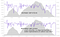# An Improved GAP Model

For our summer Strava Jam I used our new bulk access activity stream dataset to improve our current GAP (Grade Adjusted Pace) model. GAP is a running pace adjustment that corrects for the difficulty of running at different elevation gradients.

Our previous model was derived from a laboratory study  of 30 elite athletes which measured the metabolic energy cost of running at different inclines. As noted in our previous blog post on GAP, there is a known discrepancy for this GAP model in predictions of running performance on downhill gradients. Crucially, the original paper acknowledges this error as well, noting that the model accurately predicted uphill race performance, but errs on downhill race performance by as much as a factor of 3x.

We think that providing a GAP model that is accurate at predicting real world running performance can be more helpful to athletes than one predicting the metabolic energy cost of running on a treadmill. To test this hypothesis, we used a massive dataset of real world running data to construct a new model that is based on equivalent heart rate — GAP should be the pace that a runner could achieve at the same heart rate while running on level ground. This model deviates from the goal of predicting energy expenditure, but we think it more accurately models the perceived effort of running. As of today, this new GAP model is now enabled for all new runs on Strava.

From a dataset of ~6 million runs from 240 thousand athletes, having both significant elevation change and heart rate data, all 60 second windows of stream data were collected. Windows with large variances or unusual averages of heart rate, gradient, or speed were removed, and only a small fraction of windows were kept. These remaining windows exhibit smooth data in all measurements of concern, meaning that the runner was in a relatively steady state. Then the mean gradient, speed and efficiency (heartrate/speed) for each window was calculated. For each activity, window efficiencies were normalized by dividing by the median efficiency of that activity at a nearly flat gradient. This normalization reduces the variation of efficiency due to athlete fitness and other per activity variables such as temperature.

A simple statistical model was fit to this data to establish a relationship between gradient and normalized running efficiency. The model uses a variable width bucketing scheme that reflects the available quantity and variance of the data. Lines of one standard deviation bounds are also given. The running model from Minetti 2002  that Strava previously used is plotted for comparison.

The discrepancy between the models is small for positive gradients. Here the slope of the lines are almost equal, although the new model is shifted to the right by about 2%. For negative gradients the discrepancy is large, as expected. The old model predicts a minimum pace adjustment of about 0.5 at -18%, the new model predicts a minimum pace adjustment of 0.88 at -9%, and by -18% the adjustment is back to 1.0.

The new model is in much closer agreement with the intuition of most runners at Strava. In particular, anecdotal evidence from those surveyed showed that an ideal downhill running gradient would not give more than a 10% speed benefit. The old GAP model made the prediction that downhill performance would need to be up to 100% faster to count as the same flat effort.Before/after comparison of a GAP stream from a single running race.

For this Jam, I used a combination of tools:

• Spark and Mesos for the heavy lifting of processing bulk stream data
• Zeppelin for orchestrating large exploratory spark jobs on a Mesos cluster
• Bokeh and some Python in Zeppelin, for making the plot above

Although the sample size of data used in the model is extremely large and we can precisely characterize the mean of the distribution, the variance is big. With an almost unlimited amount of data, we are in the position to fit a GAP model in additional dimensions if we find other factors that correlate with gradient.

One such dimension might be effort. Instead of using heart rate to compute efficiency, we can parameterize the model over heart rate zones (or something similar). This would enable the model to account for changes in biomechanical efficiency at different speeds. For example, the metabolic cost of walking is significantly less than running at equivalent gradients. This implies that the pace adjustment factor, at large positive gradients, should be greater for low intensity running than for high intensity running.

There are some issues of the new model that I’m aware of and could work more towards addressing:

• There is a selection bias due to significantly less data for steep gradients.
• The filtering methodology imposes some further sampling bias on the data.
• The line fitting technique could almost certainly be improved.
• Using heart rate in efficiency assumes a linear relationship between heart rate and achieved pace. This assumption could be removed with further filtering by forcing all windows from each activity to have close to the same heart rate, or by some more advanced technique.
• The new model is based on typical real world running performance rather than expected metabolic energy cost. This means that the new model is better for understanding running performance, but not necessarily better at the specific goal of calculating caloric cost of running.

 Minetti, A. E. et al. (2002). Energy cost of walking and running at extreme uphill and downhill slopes. Journal of Applied Physiology 93, 1039–1046.

Written by

Written by

## Drew Robb

#### Staff Infra/Data/Geo Engineer @ Strava